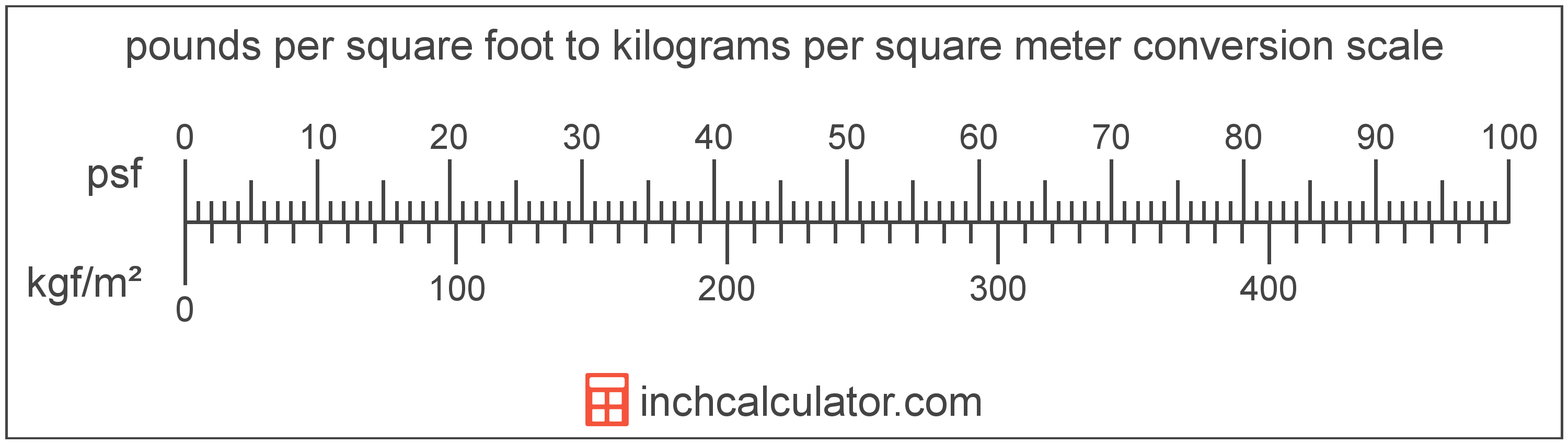# Kilograms per Square Meter to Pounds per Square Foot Converter

Enter the pressure in kilograms per square meter below to get the value converted to pounds per square foot.

Results in Pounds per Square Foot:1 kgf/m² = 0.204816 psf

Do you want to convert pounds per square foot to kilograms per square meter?

## How to Convert Kilograms per Square Meter to Pounds per Square Foot

To convert a measurement in kilograms per square meter to a measurement in pounds per square foot, multiply the pressure by the following conversion ratio: 0.204816 pounds per square foot/kilogram per square meter.

Since one kilogram per square meter is equal to 0.204816 pounds per square foot, you can use this simple formula to convert:

pounds per square foot = kilograms per square meter × 0.204816

The pressure in pounds per square foot is equal to the pressure in kilograms per square meter multiplied by 0.204816.

For example, here's how to convert 5 kilograms per square meter to pounds per square foot using the formula above.
pounds per square foot = (5 kgf/m² × 0.204816) = 1.024082 psfKilograms per square meter and pounds per square foot are both units used to measure pressure. Keep reading to learn more about each unit of measure.

## What Are Kilograms per Square Meter?

One kilogram per square meter is the pressure of equal to one kilogram-force per square meter.

The kilogram per square meter is a non-SI metric unit for pressure. A kilogram per square meter is sometimes also referred to as a kilogram per square metre or kilogram-force per meter square. Kilograms per square meter can be abbreviated as kgf/m²; for example, 1 kilogram per square meter can be written as 1 kgf/m².

In the expressions of units, the slash, or solidus (/), is used to express a change in one or more units relative to a change in one or more other units. For example, kgf/m² is expressing a change in weight relative to a change in area.

The unit is deprecated and not permitted for use with SI units.

Kilograms per square meter can be expressed using the formula:
1 kgf/m2 = 1 kgf / m2

Pressure in kilograms per square meter are equal to the kilogram-force divided by the area in square meters.

## What Are Pounds per Square Foot?

One pound per square foot is the pressure of equal to one pound-force per square foot.

The pound per square foot is a US customary and imperial unit of pressure. Pounds per square foot can be abbreviated as psf; for example, 1 pound per square foot can be written as 1 psf.

PSF can be expressed using the formula:
1 psf = 1 lbf / ft2

Pressure in pounds per square foot are equal to the pound-force divided by the area in square feet.

## Kilogram per Square Meter to Pound per Square Foot Conversion Table

Table showing various kilogram per square meter measurements converted to pounds per square foot.
Kilograms Per Square Meter Pounds Per Square Foot
1 kgf/m² 0.204816 psf
2 kgf/m² 0.409633 psf
3 kgf/m² 0.614449 psf
4 kgf/m² 0.819265 psf
5 kgf/m² 1.0241 psf
6 kgf/m² 1.2289 psf
7 kgf/m² 1.4337 psf
8 kgf/m² 1.6385 psf
9 kgf/m² 1.8433 psf
10 kgf/m² 2.0482 psf
11 kgf/m² 2.253 psf
12 kgf/m² 2.4578 psf
13 kgf/m² 2.6626 psf
14 kgf/m² 2.8674 psf
15 kgf/m² 3.0722 psf
16 kgf/m² 3.2771 psf
17 kgf/m² 3.4819 psf
18 kgf/m² 3.6867 psf
19 kgf/m² 3.8915 psf
20 kgf/m² 4.0963 psf
21 kgf/m² 4.3011 psf
22 kgf/m² 4.506 psf
23 kgf/m² 4.7108 psf
24 kgf/m² 4.9156 psf
25 kgf/m² 5.1204 psf
26 kgf/m² 5.3252 psf
27 kgf/m² 5.53 psf
28 kgf/m² 5.7349 psf
29 kgf/m² 5.9397 psf
30 kgf/m² 6.1445 psf
31 kgf/m² 6.3493 psf
32 kgf/m² 6.5541 psf
33 kgf/m² 6.7589 psf
34 kgf/m² 6.9638 psf
35 kgf/m² 7.1686 psf
36 kgf/m² 7.3734 psf
37 kgf/m² 7.5782 psf
38 kgf/m² 7.783 psf
39 kgf/m² 7.9878 psf
40 kgf/m² 8.1927 psf

## References

1. National Institute of Standards and Technology, NIST Guide to the SI, Chapter 6: Rules and Style Conventions for Printing and Using Units, https://www.nist.gov/pml/special-publication-811/nist-guide-si-chapter-6-rules-and-style-conventions-printing-and-using